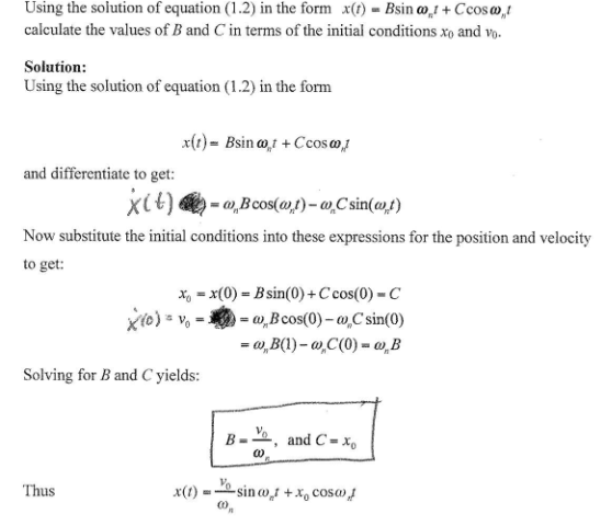Using the solution of equation in the form x(t)=Bsinwt + Ccoswt calculate the values of B and C in terms of the initial conditions x0 and v0.Using the solution of equation in the form x(t)=Bsinwt + Ccoswt calculate the values of B and C in terms of the initial conditions x0 and v0.

Vibrations Page 1 vibrations vibrations vibrations vibrations vibrations vibrations vibrations Vibrations Page 2